# Thread: Intergrating Factors

1. ## Intergrating Factors

Been struggling to get started on this for hours.
The temperature of an object at time t is found from the equation
$\displaystyle \frac{d\theta}{dt}$+ 0.1θ = 5 - 2.5t
Given that when t=0, temperature is 60˚C. find the temperature when t=2 seconds
Any advice on getting started much appreciated.
Thanks

2. Just in case a picture helps...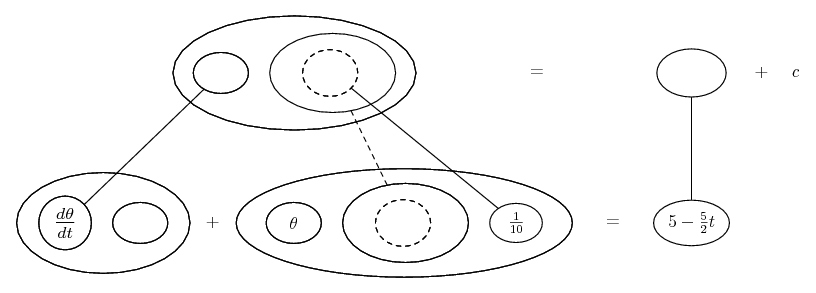... where the chain rule...... is wrapped inside the product rule...... (legs un-crossed version). Straight continuous lines differentiate downwards (integrate up) with respect to x, and the straight dashed line similarly but with respect to the dashed balloon expression (the inner function of the composite which is subject to the chain rule).

Now fill out the rest of the bottom row, and you'll have an exact product-rule derivative on the left-hand side, and you can integrate both sides with respect to t.

The general drift is...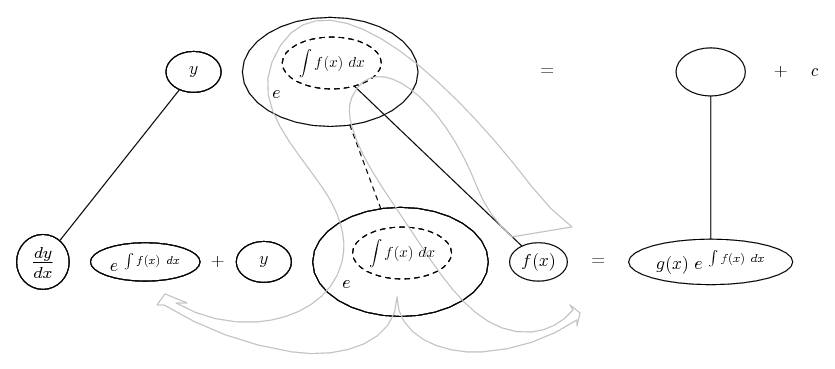And the rest...

Spoiler: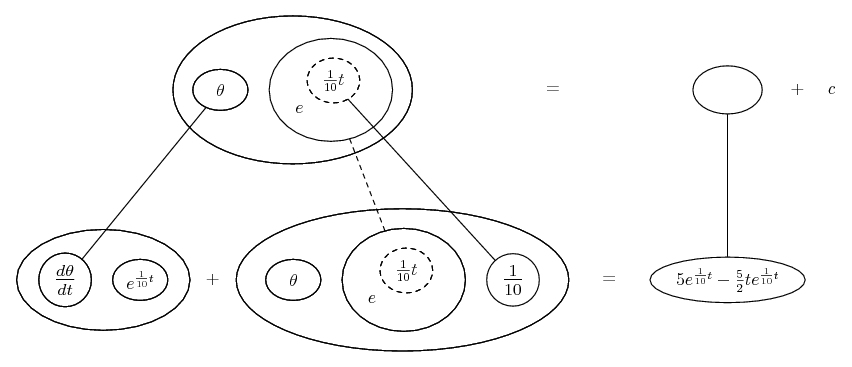Spoiler: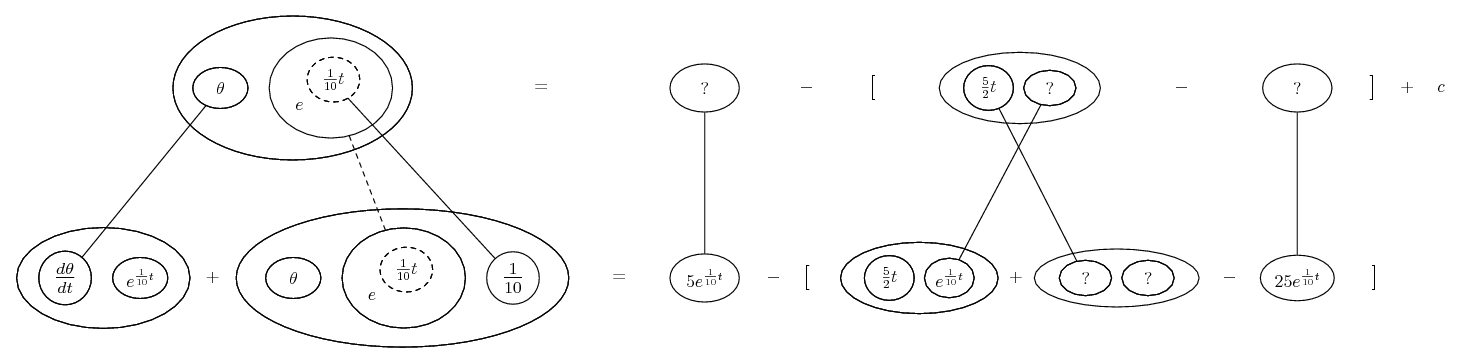_________________________________________
Don't integrate - balloontegrate!

Balloon Calculus; standard integrals, derivatives and methods

Balloon Calculus Drawing with LaTeX and Asymptote!

3.Originally Posted by jamm89Been struggling to get started on this for hours.
The temperature of an object at time t is found from the equation
$\displaystyle \frac{d\theta}{dt}$+ 0.1θ = 5 - 2.5t
Given that when t=0, temperature is 60˚C. find the temperature when t=2 seconds
Any advice on getting started much appreciated.
Thanks
You know you can solve this by the integrating factor method, so what did you try?

4. I have got this far but not sure what to do next.
$\displaystyle \frac{d\theta}{dt}$e^0.1t = 5e^0.1t - 2.5e^0.1t
Thanks

5. Either you're hoping you've integrated already in which case why the differentials(?), or you're hoping you're ready to integrate, having multiplied through by the I.P - in which case, you've dropped a t on the RHS, and you didn't really grasp the method. Note the bottom rows of my pictures are the situation before integrating, which wants to be...

$\displaystyle \frac{d \theta}{d t} e^{\frac{1}{10} t} + \frac{1}{10} \theta\ e^{\frac{1}{10} t} = 5 e^{\frac{1}{10} t} - \frac{5}{2} t e^{\frac{1}{10} t}$

The right-hand integration will involve 'parts', for which you could assign u du and v dv as usual or, if you were feeling adventurous enough to consult my pic above, you might also want to consider the general drift as...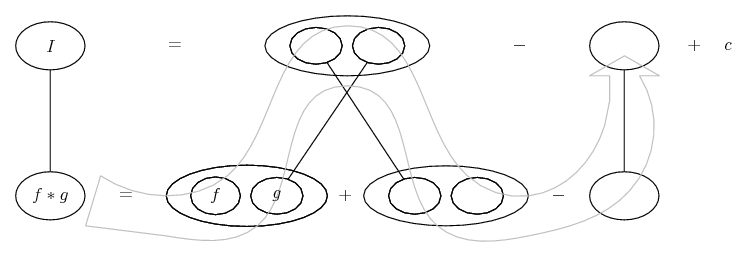But more orthodox advice will be available too...

6. Here is what i have got but i cant get the right answerIntergral (5-2.5t) e^t/10 dx

By parts
u = 5-2.5t dv/dx = e^t/10
du/dx = -2.5 v = 10e^t/10

= 10e^t/10 . 5-2.5t - intergral 10e^t/10 . -2.5
= 50e^t/10 - 25te^t/10 - intergral -25e^t/10
Intergrate again
= 50e^t/10 - 25te^t/10 - (-250e^t/10) + c

But this does not give me the answer of 60 when i make t=0Can someone show me where i have gone wrong please. I have tried to used the table below but struggled.
Thanks

7.Originally Posted by jamm89But this does not give me the answer of 60 when i make t=0It isn't meant to! Use the fact that the temperature is 60 when t=0 to determine the value of c. Then you have a complete formula for finding temperatures from t's.

8.Originally Posted by tom@ballooncalculusIt isn't meant to! Use the fact that the temperature is 60 when t=0 to determine the value of c. Then you have a complete formula for finding temperatures from t's.
So i have plugged t=0 in and it gives me
60=50-0+250 +c
60=300 + c
c = -240

Then i put t=2
and i get
61.07-61.07+305.35 - 240
= 65.35

9.Originally Posted by jamm89So i have plugged t=0 in and it gives me
60=50-0+250 +c
60=300 + c
c = -240
GoodThen i put t=2
and i get
61.07-61.07+305.35 - 240
= 65.35Show steps...

10. 50e^t/10 - 25te^t/10 - (-250e^t/10) + c
I substituted t=2 and c=-240
Which gives
50e^(2/10) - ((25x2)(e^2/10))- (-250xe^2/10) -240
61.07 - 61.07 - (-305.35) - 240
=65.35

Is that correct?

11. You've only subbed into the RHS. Thought the LHS was theta on its own? Only when t was zero!

12. So what do i need to do to be able to substitute in to get theta on its own?
Thanks

13. You can sub straight in already, just on the LHS you'll have theta times a constant, and I'm sure you'll be able to deal with that - but it's a while since you showed exactly what you've arrived at, so we might be at cross purposes. Show the whole equation.

14. The temperature of an object at time t is found from the equation+ 0.1θ = 5 - 2.5t
Given that when t=0, temperature is 60˚C. find the temperature when t=2 seconds

p (t) = 0.1
Q (t) = 5-2.5t
If = e^t/10e^t/10 + 0.1θe^t/10 = 5e^t/10 - 2.5te^t/10

By parts

$\displaystyle \int{(5-2.5t) e^ {t/10}}$
u = 5-2.5t dv/dx = $\displaystyle e^ {t/10}$
du/dx = -2.5 v = $\displaystyle 10e^ {t/10}$
$\displaystyle \int{(5-2.5t) e^ {t/10}dx} = 10e^ {t/10}(5-2.5t) - \int{10e^{t/10}. -2.5}$

Substitute in t = 0
$\displaystyle =50e^{t/10} - 25te^{t/10} - \int{-25e^{t/10}}$
$\displaystyle =50e^{t/10} - 25te^{t/10} - (-250e^{t/10}) + c$
= 50 - 0 + 250 + c

substitute 60 in to give c
60 = 300 + c
c = - 240
Now im not sure what to do from there.
Thanks

15. All I meant by the 'whole equation' was (not the whole of your working to date but just...)

$\displaystyle 50\ \theta\ e^{t/10}\ =\ 50e^{t/10} - 25te^{t/10} - (-250e^{t/10}) - 240$

(or simpler version).

Sub into that. You do see where it came from? We integrated both sides of the differential equation (after multiplying through by the I.F.) with respect to t. (In my pictures the bottom row is the differential equation.) For the RHS we needed parts, while on the left we had an exact product-rule derivative.

#### Search Tags

factors, intergrating